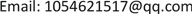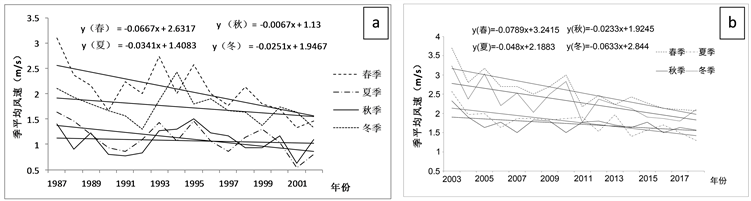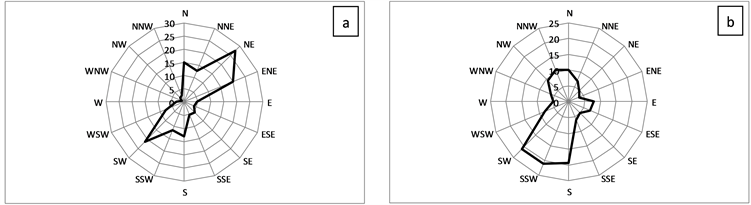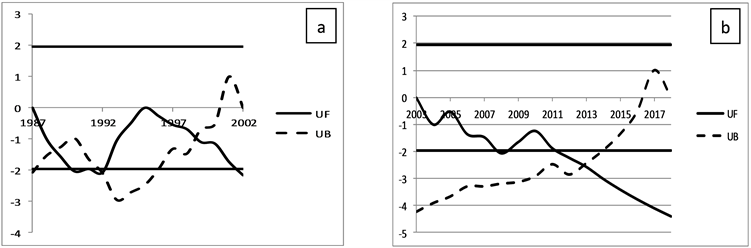﻿ 盘州市气象站迁站前后风资料对比分析 Comparative Analysis on Wind Data of Transfer of Panzhou Meteorological

Climate Change Research Letters
Vol. 08  No. 06 ( 2019 ), Article ID: 32848 , 7 pages
10.12677/CCRL.2019.86083

Comparative Analysis on Wind Data of Transfer of Panzhou Meteorological

Xuda Wang1, Yanmei Zhang2, Guiqiong Li1, Lin Hu3

1Panzhou Meteorology Bureau, Panzhou Guizhou

2Guizhou Province Observatory, Guiyang Guizhou

3Meteorological Bureau of Dushan, Dushan GuizhouReceived: Oct. 15th, 2019; accepted: Oct. 30th, 2019; published: Nov. 6th, 2019ABSTRACT

By using the daily wind speed observation data from 1987 to 2018 in the old and new Panzhou meteorological station, gale days, average wind speed and wind direction frequency of annual maximum wind speed in new and old Panzhou stations were analyzed. The results show that the number of gale days in the old and new Panzhou stations is decreasing, the number of gale days in the 16 years before relocation is only 200 days, the number of gale days in the 16 years after relocation is increasing obviously, and the number of gale days reaches 366 days; the average wind speed of the old and new Panzhou stations in every year and season is also decreasing, especially in spring. The main wind speed is NE, ENE and SW; the maximum wind speed is SW, SSW, S and SW. The annual average wind speed of the new station changed abruptly in 1992 and 1998, and a sudden break occurred in the old station in 2013.

Keywords:Panzhou, the Wind Speed, the Direction of the Wind, Comparison and Analysis

1贵州省盘州市气象局，贵州 盘州

2贵州省气象台，贵州 贵阳

3贵州省独山县气象局，贵州 独山1. 引言

2. 研究资料与方法

2.1. Mann-Kendall突变检测风速的突变

Mann-Kendall检验法最初是由H.B. Mann和M.K. Kendall提出并适用于气象、水文等非正态分布检验的一种方法  ，利用Mann-Kendall检验法将盘州市1987~2018年的平均风速进行显著性检验，具体检验方法如下：

${S}_{k}={\sum }_{i=1}^{k}{r}_{i},\text{\hspace{0.17em}}\text{\hspace{0.17em}}k=2,3,\cdots ,n$

${r}_{i}=\left\{\begin{array}{l}+1,\text{\hspace{0.17em}}\text{\hspace{0.17em}}\text{\hspace{0.17em}}\text{ }\text{ }当{x}_{i}>{x}_{j}\\ 0,\text{\hspace{0.17em}}\text{\hspace{0.17em}}\text{\hspace{0.17em}}\text{\hspace{0.17em}}\text{\hspace{0.17em}}当{x}_{i}\le {x}_{j}\end{array}\text{\hspace{0.17em}}\text{\hspace{0.17em}}\text{\hspace{0.17em}}\text{\hspace{0.17em}}j=1,2,\cdots ,i$

$U{F}_{k}=\frac{\left[{s}_{k}-E\left({s}_{k}\right)\right]}{\sqrt{\mathrm{var}\left({s}_{k}\right)}},\text{\hspace{0.17em}}\text{\hspace{0.17em}}k=1,2,\cdots ,n$

$\left\{\begin{array}{l}E\left({s}_{k}\right)=\frac{k\left(k-1\right)}{4}\\ \mathrm{var}\left({s}_{k}\right)=\frac{k\left(k-1\right)\left(2k+5\right)}{72}\end{array}\text{\hspace{0.17em}}\text{\hspace{0.17em}}\text{\hspace{0.17em}}k=2,3,\cdots ,n$

$U{F}_{i}$ 就是标准正态分布，通过显著性检验，若 $|U{F}_{i}|>{U}_{a}$，可以说明盘州市的年平均风速存在着明显的趋势变化，根据上式，将时间序列x从后往前，逆序 ${x}_{n},{x}_{n-1},\cdots ,{x}_{1}$，再次计算，并且使得出 $U{B}_{k}=-U{F}_{k}\left(k=n,n-1,\cdots ,1\right)$$U{B}_{1}=0$，通过两次的运算便可得到突变的时间定格在哪一年。

2.2. 线性倾向法检验

${X}_{i}=a+b{t}_{i},\text{\hspace{0.17em}}\text{\hspace{0.17em}}i=1,2,\cdots ,n$

3. 结果与分析

3.1. 盘州市新旧站址大风日数年际变化对比分析Figure 1. Contrastive analysis of gale days in Panzhou new station from 1987 to 2002 (a) and old station from 2003 to 2018 (b)

3.2. 盘州市新旧站址平均风速变化对比分析Figure 2. The annual average wind speed change trend of the old station (a) in Panzhou from 1987 to 2002 and the new station (b) from 2013 to 2018

3.3. 盘州市新旧站址平均风速的季节变化对比分析Figure 3. Linear trend change of monsoon mean wind speed in Panzhou old station (a) from 1987 to 2002 and new station (b) from 2003 to 2018

3.4. 最大风速的风向频率特征对比分析Figure 4. Rose Charts of maximum wind speed frequency in Panzhou old station (a) from 1987 to 2002 and new station (b) from 2003 to 2018

3.5. 盘州市新旧站址的平均风速的突变分析Figure 5. Mann-Kendall curves of old station site (a) from 1987 to 2002 and new station site (b) from 2003 to 2018 in Panzhou

4. 环境对气象要素的影响讨论及主要结论

4.1. 环境对风资料的影响讨论

4.2. 主要结论

1) 盘州市新旧站址的各季平均风速均呈减少趋势，旧站址春季的平均风速减少最为明显，冬季、夏季和秋季减小的趋势不明显；新站址16a春季的平均风速最大，其次是冬季，夏季和秋季的平均风速相对于春冬两季较小。盘州市新旧站址春季的平均风速最大，冬季的平均风速次之，夏季和秋季的平均风速较为平稳，减小的趋势也不明显。

2) 盘州市旧站址最大风速主要常出现在东北风(NE)、东北偏东风(ENE )、西南风(SW)这三个方向上，其中出现次数最多的方向为东南(NE)方向。而新站址的16年里盘州市最大风速主要出现在西南风(SW)、西南偏南风(SSW)、偏南风(S)这三个方向上，其中出现次数最多的方向为西南(SW)方向，说明盘州市最大风速出现的风向因地理位置的不同而发生了一定的变化。

3) 盘州市1987~2018年，盘州市年平均风速有下降的趋势，从2010年以后下降趋势明显，并分别在1992年、1998年和2013年发生过一次风速突变，在1998年风速突变明显。

Comparative Analysis on Wind Data of Transfer of Panzhou Meteorological[J]. 气候变化研究快报, 2019, 08(06): 762-768. https://doi.org/10.12677/CCRL.2019.86083

1. 1. 李泽椿, 朱荣, 何晓风, 等. 风能资源评估技术方法研究[J]. 气象学报, 2007, 65(5): 708-715.

2. 2. 谢任波, 舒国勇, 晏理华, 等. 近40a铜仁地区雨量和雨日的变化特征[J]. 贵州气象, 2011(4): 29-31.

3. 3. 王旭达, 张艳梅, 陈海涛. 贵州省盘县近50 a降水特征分析[J]. 贵州气象, 2016(6): 37-41.

4. 4. 李悦佳, 贺新光, 卢希安, 谭子芳. 1960-2015年长江流域风速的时空变化特征[J]. 热带地理, 2018, 38(5): 660-667.

5. 5. 易志学, 徐德志. 1957-2010年黎平县气候变化特征分析[J]. 贵州气象, 2012(4): 27-29.

6. 6. 魏凤英. 现代气候统计诊断与预测技术[M]. 第2版. 北京: 气象出版社, 2007: 63-66.

7. 7. Sheng, Y., Pilona, P. and Cavadiasb, G. (2002) Power of the Mannk-Kendall and Spearman’s Rho Tests for Detecting Monotonic Trends in Hydrological Series. Journal of Hydrolo-gy, 259, 256-270.

8. 8. 李志坤, 张风丽, 王国军, 等. 北京市1993-2011年风速变化与下垫面粗糙特性关系研究[J]. 测绘通报, 2017(12): 29-32.

9. 9. 杨静, 汪超, 雷云, 等. 春季西南热低压的发生发展及结构特征[J]. 气象, 2013, 39(2): 146-155.